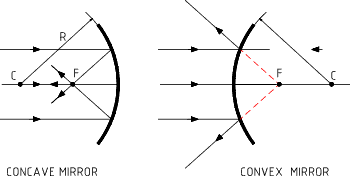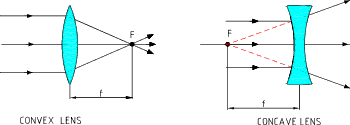Mirrors - Lenses
 Mirrors & Lenses Spherical Mirrors Spherical Mirrors can be either Concave or Convex. The focal length (f) is the distance from the mirror face to the focal point (F) where the reflected rays or parallel light meet.   For a spherical mirror with a surface radius R. Concave mirror f = + R/2 Concave mirror f = - R/2   Note: for the convex mirror the focal point is a virtual point located behind the surface of the mirror.Mirror Equation For an object located a distance p from a mirror the image is located at a distance q from the mirror surface as difined below: 1 / p + 1 / q = 1 / f Therefore : p = q.f / ( q - f )   and   q = p.f / ( p-f )   and   f = p.q / ( p + q ) Magnification The linear magnification ( m ) of any optical system is the ratio between the size (height or width or other transverse dimension ) of image and the associated size of the relevant object.  In the case of a mirror. m = h' / h = - q / p The magnification = Image Height/ Object Height = image distance / object distance. A positive magnification indicates a image in the same direction as the object.   A negative image indicates a inverted image... Lenses These notes related only to simple thin lenses with neglible glass thickness... A lens is defined by the two surface radii ( R1 & R2 ) and its focal length(f)    for a convex lens the focal point (F) is a virtual focal point..The equation is used to calculate the focal length 1 / f = ( n - 1 ) . ( 1 / R1 + 1 / R2 ) n = the index of refraction of the lens material relative to the surrounding medium. R1 and R2 are positive for convex lens and negative for concave lens The lens equation The object distance, the image distance and the focal length of a lens are related by the following formula. 1 / f = 1 /p + 1 / q Therefore p = q.f / (q -f)    and   q = p . f / ( p - f )   and   f = p . q. / ( p + q ) Magnification... The same as for mirrors as indicated above.. The linear magnification ( m ) of any optical system is the ratio between the size (height or width or other transverse dimension ) of image and the associated size of the relevant object.  In the case of a mirror. m = h' / h = - q / p The magnification = Image Height/ Object Height = image distance / object distance. A positive magnification indicates a image in the same direction as the object.   A negative image indicates a inverted image...

### Sites & Links for physics of lens and mirrors

1. ....Short set of notes with relevant formula and pictorial views
2. GCSE Physics - Waves....Lots of very pretty and informative tutorials
3. Mirrors and lenses chapter 38....U of Nebraska- A very informative document download on Mirrors and Lenses
4. PhysicsClassroom Lens ....Easy to follow Notes